# TeimOrbit 2D Road Type: sine_sweep

A sine-shaped road profile with slowly varying frequency and amplitude is calculated in two different ways:

## Linear Sweep

The frequency increases linearly with respect to travel distance. The road height value z(s) as function of travel distance s is calculated as follows: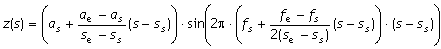Note: The factor 2 in the denominator is not an error!

The actual frequency (= derivative of the sine function argument with respect to travel path, divided by 2π; this is not equal to the factor that is multiplied by 2π(s-ss) in the sine function!) is given by: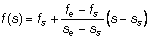## Logarithmic sweep

With every cycle, the wave length decreases by a constant factor. The road height value is calculated as follows: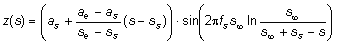Where,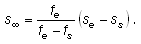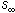is the travel path where theoretically an infinitely high frequency is reached, measured relative to sweep start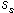. The actual frequency is given by: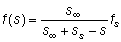The swept sine road is implemented as a 2D road type. If the ROAD_TYPE is set to sine_sweep, COSIN/road searches and reads the following data items in the [PARAMETERS] section:
Name of Input Variable Unit Meaning
start length Start of swept sine wave (travel distance).
end length End of swept sine wave (travel distance).
amplitude_at_start length Amplitude of swept sine wave at start.
amplitude_at_end length Amplitude of swept sine wave at end.
wave_length_at_start length Wave length of swept sine wave at start.
wave_length_at_end length Wave length of swept sine wave at end. Must be less than or equal to wave_length_at_start.
sweep_type 0/1
0
Frequency changes linearly with respect to travel distance.
1
Wave length changes each cycle by a constant factor.